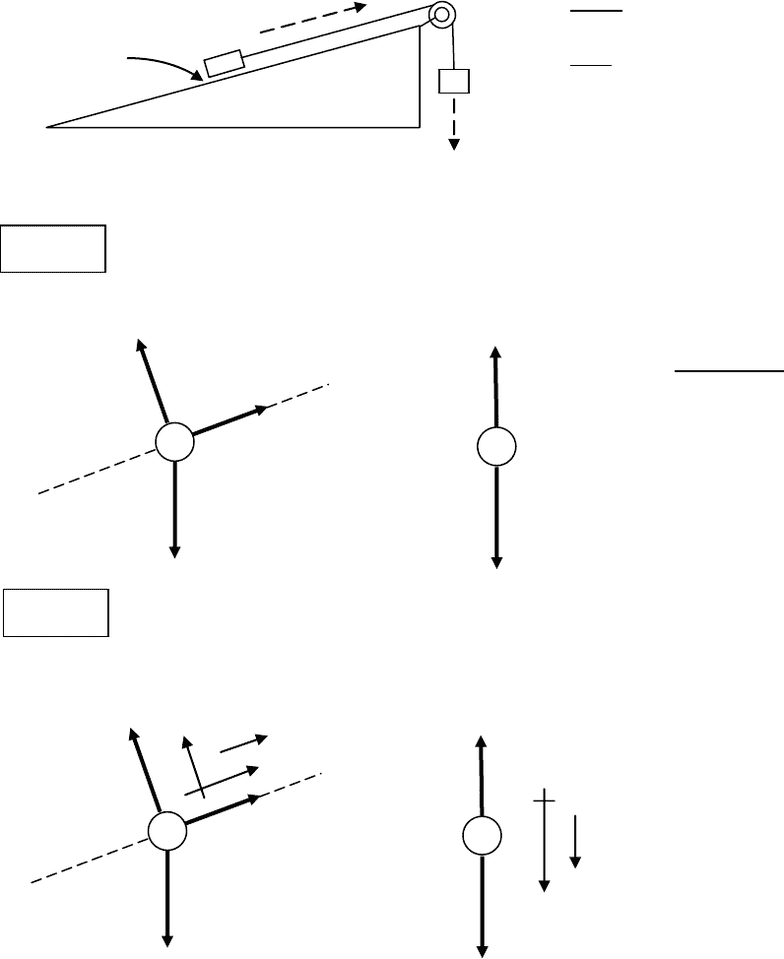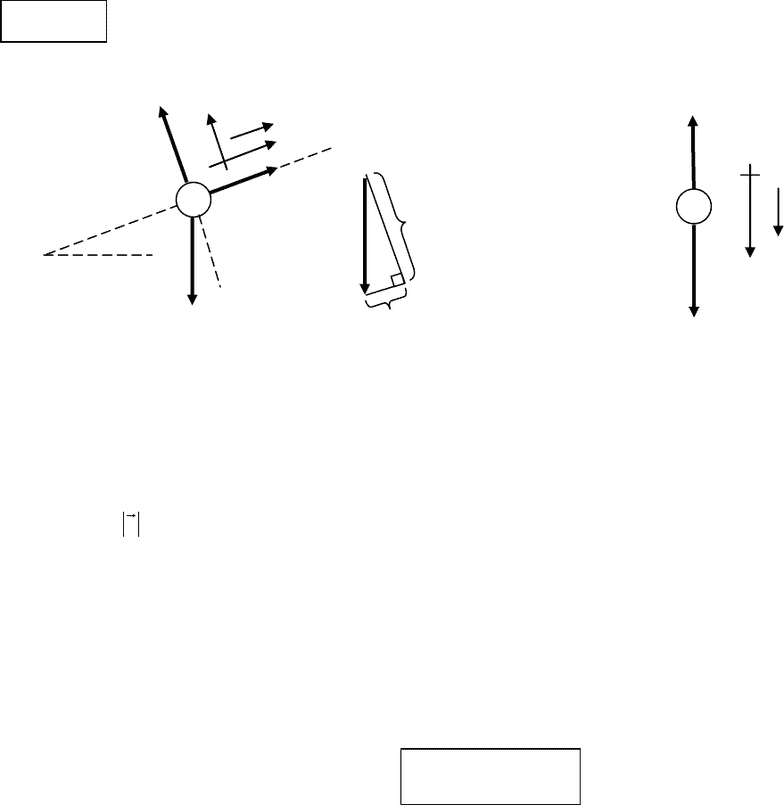Class Notes (1,100,000)
US (480,000)
CU-Boulder (3,000)
PHYS (100)
Marino (5)
Lecture 5

# PHYS 1110 Lecture Notes - Lecture 5: Acceleration, Centripetal Force, Centrifugal Force

Department
Physics
Course Code
PHYS 1110
Professor
Marino
Lecture
5

This preview shows pages 1-3. to view the full 9 pages of the document.NA-1
Some Applications of Newton’s Laws.
In this chapter, we get some practice applying Newton’s laws to various physical problems. We
do not introduce any new laws of physics.
Solving Fnet = ma problems with multiple bodies
Problem: A mass m1 is pulled up a frictionless incline by a string over a pulley and a hanging
mass m2.
We know m1, m2, and the angle .
We seek :
T = tension in the cord,
a = acceleration of the mass,
N = normal force on m1
Step 1
Draw a free-body diagram for each moving object.
Label the force arrows with
the magnitudes of the forces.
Notice that T is the same for
both objects (by NIII).
Use m1, m2, in the diagrams,
not m.
Step 2
For each object, choose xy axes so that the acceleration vector a is in the (+) direction.
Notice that we can choose
different axes for the different
objects. And we can tilt the
axes if necessary.
m1
m2
no friction
T
N
m1g
T
m2g
T
N
T
m2g
+x
+y
a
+y
a

Only pages 1-3 are available for preview. Some parts have been intentionally blurred.NA-2
Step 3 For each object, write the equations
x x y y
F m a , F m a

m1 :
11
1
x-eqn (1) T m gsin m a
y-eqn (2) N m gcos 0
 
 
m2 :
22
(3) m g T m a  
Notice that
aa
is the same for both m1 and m2 since they are connected by a string that
doesn't stretch.
Now we have a messy algebra problem with 3 equations in 3 unknowns:
11
1
22
(1) T m gsin m a
(2) N m gcos 0
(3) m g T m a
 
 
 
The unknowns are T , N , and a.
We can solve for N right away. Eqn (2)
1
N m gcos
Now we have 2 equations [(1) & (3)] in 2 unknowns ( a & T ).
Solve (1) for T and plug into (3) to get an equation without an T :
(1)
1 1 1
T m gsin m a m (gsin a)    
(3)
2 1 2
m g [m (gsin a)] m a  
T
N
T
m2g
x
y
+y
a
m1g
m1g cos
m1g sin
2
1
a

Only pages 1-3 are available for preview. Some parts have been intentionally blurred.NA-3
Now solve this last equation for a:
Finally, if you have any strength left, we can solve for tension T by plugging our expression for
acceleration a back into either (1) or (3) and solving for T. From (3), we have
2 2 2
T m g m a m (g a)  
.
Plugging in our big expression for a, we get
21
22
12
m g m gsin
T m (g a) m g (m m )


 


We can simplify:
1 2 2 1 1 2 2 1
22
1 2 1 2 1 2
1 1 2 1
2
1 2 1 2
g(m m ) m g m gsin m g m g m g m gsin
T m m
(m m ) (m m ) (m m )
m g m gsin m m g
T m T (1 sin )
(m m ) (m m )
 
  
 
 
 
 


 



Do these expressions make sense? Let’s check some limits.
If m1 = 0, then m2 should be freely falling with a = g and the tension T should be zero. Check
that this is so.
If m2 = 0, then m1 should slide down the incline with acceleration a = g sin (since it would be
accelerating in the negative direction). Check that this is so.
2 1 1 2 1 2
21
12
m g m gsin m a m a a(m m )
m g m gsin
a(m m )
  
